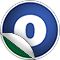# KMedoids

### Related Docs: class KMedoids | package cluster

#### object KMedoids extends Logging with Serializable

Utilities used by K-Medoids clustering

Linear Supertypes
Serializable, Serializable, Logging, AnyRef, Any
Ordering
1. Alphabetic
2. By Inheritance
Inherited
1. KMedoids
2. Serializable
3. Serializable
4. Logging
5. AnyRef
6. Any
1. Hide All
2. Show All
Visibility
1. Public
2. All

### Value Members

1. #### final def !=(arg0: Any): Boolean

Definition Classes
AnyRef → Any
2. #### final def ##(): Int

Definition Classes
AnyRef → Any
3. #### final def ==(arg0: Any): Boolean

Definition Classes
AnyRef → Any
4. #### def apply[T](metric: (T, T) ⇒ Double): KMedoids[T]

Return a KMedoids object with the given metric function, and other parameters defaulted.

Return a KMedoids object with the given metric function, and other parameters defaulted.

Defaults are as follows:

k = 2

maxIterations = 25

epsilon = 0.0

fractionEpsilon = 0.0001

sampleSize = 1000

seed = randomly initialized seed value

metric

The metric function to impose on elements of the data space

5. #### final def asInstanceOf[T0]: T0

Definition Classes
Any
6. #### def clone(): AnyRef

Attributes
protected[java.lang]
Definition Classes
AnyRef
Annotations
@throws( ... )
7. #### final def eq(arg0: AnyRef): Boolean

Definition Classes
AnyRef
8. #### def equals(arg0: Any): Boolean

Definition Classes
AnyRef → Any
9. #### def finalize(): Unit

Attributes
protected[java.lang]
Definition Classes
AnyRef
Annotations
@throws( classOf[java.lang.Throwable] )
10. #### final def getClass(): Class[_]

Definition Classes
AnyRef → Any
11. #### def hashCode(): Int

Definition Classes
AnyRef → Any
12. #### final def isInstanceOf[T0]: Boolean

Definition Classes
Any
13. #### def logDebug(msg: ⇒ String): Unit

Attributes
protected
Definition Classes
Logging
14. #### def logError(msg: ⇒ String): Unit

Attributes
protected
Definition Classes
Logging
15. #### def logInfo(msg: ⇒ String): Unit

Attributes
protected
Definition Classes
Logging
16. #### def logWarning(msg: ⇒ String): Unit

Attributes
protected
Definition Classes
Logging
17. #### def logger: Logger

Definition Classes
Logging
18. #### final def ne(arg0: AnyRef): Boolean

Definition Classes
AnyRef
19. #### final def notify(): Unit

Definition Classes
AnyRef
20. #### final def notifyAll(): Unit

Definition Classes
AnyRef
21. #### def sampleBySize[T](data: Seq[T], sampleSize: Int): Seq[T]

Return a random sample of data having an expected sample size of the requested amount.

Return a random sample of data having an expected sample size of the requested amount.

data

The input data to sample

sampleSize

The desired sample size.

returns

A sample whose expected mean size is sampleSize.

22. #### def sampleBySize[T](data: Seq[T], sampleSize: Int, seed: Long): Seq[T]

Return a random sample of data having an expected sample size of the requested amount.

Return a random sample of data having an expected sample size of the requested amount.

data

The input data to sample

sampleSize

The desired sample size.

seed

Seed for RNG

returns

A sample whose expected mean size is sampleSize.

23. #### def sampleBySize[T](data: RDD[T], sampleSize: Int): Seq[T]

Return a random sample of data having an expected sample size of the requested amount.

Return a random sample of data having an expected sample size of the requested amount.

data

The input data to sample

sampleSize

The desired sample size.

returns

A sample whose expected mean size is sampleSize.

24. #### def sampleBySize[T](data: RDD[T], sampleSize: Int, seed: Long): Seq[T]

Return a random sample of data having an expected sample size of the requested amount.

Return a random sample of data having an expected sample size of the requested amount.

data

The input data to sample

sampleSize

The desired sample size.

seed

Seed for RNG

returns

A sample whose expected mean size is sampleSize.

25. #### def sampleDistinct[T](data: Seq[T], k: Int): Seq[T]

Return a given number of distinct elements randomly selected from data

Return a given number of distinct elements randomly selected from data

data

The data to sample from

k

The number of distinct samples to return

returns

A collection of k distinct elements randomly selected from the data

Note

If the number of distinct elements in the data is < k, an exception will be thrown

26. #### def sampleDistinct[T](data: Seq[T], k: Int, seed: Long): Seq[T]

Return a given number of distinct elements randomly selected from data

Return a given number of distinct elements randomly selected from data

data

The data to sample from

k

The number of distinct samples to return

seed

A seed to use for RNG when sampling

returns

A collection of k distinct elements randomly selected from the data

Note

If the number of distinct elements in the data is < k, an exception will be thrown

27. #### def sampleDistinct[T](data: Seq[T], k: Int, rng: Random): Seq[T]

Return a given number of distinct elements randomly selected from data

Return a given number of distinct elements randomly selected from data

data

The data to sample from

k

The number of distinct samples to return.

rng

Random number generator to use when sampling

returns

A collection of k distinct elements randomly selected from the data

Note

If the number of distinct elements in the data is < k, an exception will be thrown

28. #### def sampleFraction[N](n: N, sampleSize: Int)(implicit arg0: Numeric[N]): Double

Return the random sampling fraction corresponding to a desired number of samples

Return the random sampling fraction corresponding to a desired number of samples

n

The size of data being sampled from

sampleSize

The desired sample size

returns

A sampling fraction, >= 0.0 and <= 1.0 that will yield the desired sample size

Note

When used with typical Bernoulli sampling the returned samping fraction will yield a sample size that varies randomly, with a mean of 'sampleSize'

29. #### final def synchronized[T0](arg0: ⇒ T0): T0

Definition Classes
AnyRef
30. #### def toString(): String

Definition Classes
AnyRef → Any
31. #### final def wait(): Unit

Definition Classes
AnyRef
Annotations
@throws( ... )
32. #### final def wait(arg0: Long, arg1: Int): Unit

Definition Classes
AnyRef
Annotations
@throws( ... )
33. #### final def wait(arg0: Long): Unit

Definition Classes
AnyRef
Annotations
@throws( ... )